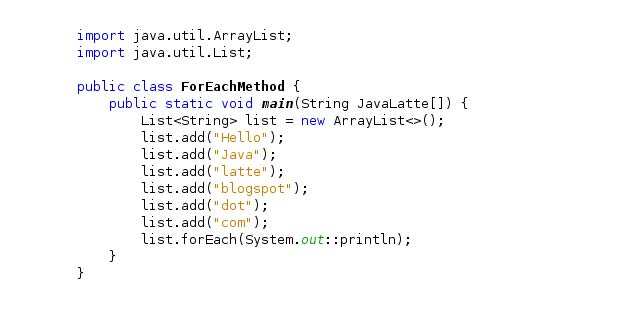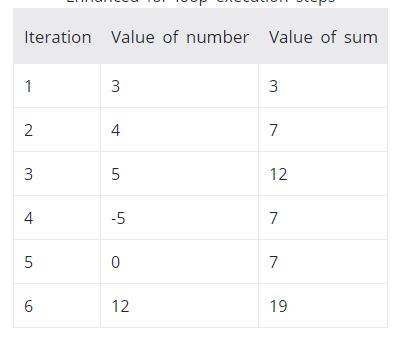# If you are working with arrays and sets, you can use another for loop structure (advanced for loop form) to duplicate their items. This type of loop is called for-each because the loop is repeated through each array / set element.

Here is an example of repeating elements of an array using the standard for loop:

1. class ForLoop {
2. public static void main (String [] args) {
3. char [] vowels = {‘a’, ‘e’, ​​’i’, ‘o’, ‘u’};
4. for (int i = 0; i <vowels.length; ++ i) {
5. System.out.println (vowels [i]);
6. }
7. }
8. }

You can also write the above code using the for-each loop:

1. class AssignmentOperator {
2. public static void main (String [] args) {
3. char [] vowels = {‘a’, ‘e’, ​​’i’, ‘o’, ‘u’};
4. // foreach loop
5. for (char item: vowels) {
6. System.out.println (item);
7. }
8. }
9. }

The output of both codes is similar and equal to:

1. a
2. e
3. i
4. o
5. u

Using the advanced for loop is easier to write and makes the code more readable. Hence it is usually recommended more than the standard form.

## For-each ring structure

First let’s look at the for-each loop structure:

for (data_type item: collection) {

}

In the above structure,

• collection is a collection or array on which you want to write a circle.
• item is a single element of the collection.

## How does the for-each loop work?

Here’s how the for-each loop works.

• Repeat through any element in the given array or collection,
• Stores each item in an item.
• And executes the body of the ring.

Example: for-each loop

The following program calculates the sum of all the elements of an array of integers.

1. class EnhancedForLoop {
2. public static void main (String [] args) {
3. int [] numbers = {3, 4, 5, -5, 0, 12};
4. int sum = 0;
5. for (int number: numbers) {
6. sum + = number;
7. }
8. System.out.println (“Sum =” + sum);
9. }
10. }

Output

Sum = 19

In the above program, the execution of the for-each loop is as follows:You see the repetition of the for-each loop

• All elements of numbers are repeated.
• Each element is stored in the number variable.
• The body of the loop is executed, ie the number is added to the sum.